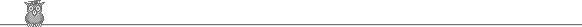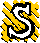### 25th Putnam 1964A1.  Let A1, A2, A3, A4, A5, A6 be distinct points in the plane. Let D be the longest distance between any pair, and d the shortest distance. Show that D/d ≥ √3.A2.  α is a real number. Find all continuous real-valued functions f :[0, 1] → (0, ∞) such that ∫01 f(x) dx = 1, ∫01 x f(x) dx = α, ∫01 x2 f(x) dx = α2.A3.  The distinct points xn are dense in the interval (0, 1). x1, x2, ... , xn-1 divide (0, 1) into n sub-intervals, one of which must contain xn. This part is divided by xn into two sub-intervals, lengths an and bn. Prove that ∑ anbn(an + bn) = 1/3.A4.  The sequence of integers un is bounded and satisfies un = (un-1 + un-2 + un-3un-4)/(un-1un-2 + un-3 + un-4). Show that it is periodic for sufficiently large n.A5.  Find a constant k such that for any positive ai, ∑1∞ n/(a1 + a2 + ... + an) ≤ k ∑1∞1/an.A6.  S is a finite set of collinear points. Let k be the maximum distance between any two points of S. Given a pair of points of S a distance d < k apart, we can find another pair of points of S also a distance d apart. Prove that if two pairs of points of S are distances a and b apart, then a/b is rational.B1.  an are positive integers such that ∑ 1/an converges. bn is the number of an which are ≤ n. Prove lim bn/n = 0.B2.  S is a finite set. A set P of subsets of S has the property that any two members of P have at least one element in common and that P cannot be extended (whilst keeping this property). Prove that P contains just half of the subsets of S.B3.  R is the reals. f : R → R is continuous and for any α > 0, limn→∞ f(nα) = 0. Prove limx→∞ f(x) = 0.B4.  n great circles on the sphere are in general position (in other words at most two circles pass through any two points on the sphere). How many regions do they divide the sphere into?B5.  Let an be a strictly monotonic increasing sequence of positive integers. Let bn be the least common multiple of a1, a2, ... , an. Prove that ∑ 1/bn converges.B6.  D is a disk. Show that we cannot find congruent sets A, B with A ∩ B = ∅, A ∪ B = D.To avoid possible copyright problems, I have changed the wording, but not the substance, of all the problems. The original text and solutions are available in: A M Gleason, R E Greenwood & L M Kelly, The William Lowell Putnam Mathematical Competition, Problems and Solutions, 1938-1964, MAA 1980. Out of print, but available in some university libraries.

Putnam home